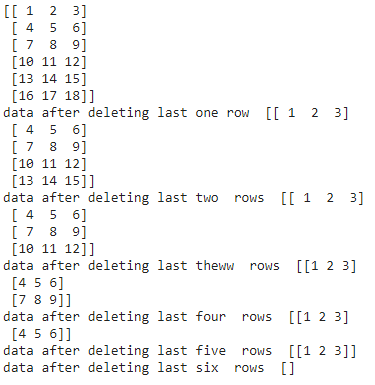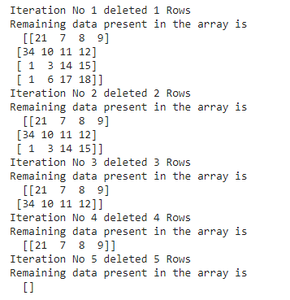# How to delete last N rows from Numpy array?

• Last Updated : 28 Apr, 2021

In this article, we will discuss how to delete the last N rows from the NumPy array.

### Method 1: Using Slice Operator

Slicing is an indexing operation that is used to iterate over an array.

Syntax: array_name[start:stop]

where start is the start is the index and stop is the last index.

We can also do negative slicing in Python. It is denoted by the below syntax.

Syntax: array_name[: -n]

where, n is the number of rows from last to be deleted.

Example1:

We are going to create an array with 6 rows and 3 columns and delete last N rows using slicing.

## Python3

 `# importing numpy module``import` `numpy as np`` ` `# create an array with 6 rows and 3 columns``a ``=` `np.array([[``1``, ``2``, ``3``], [``4``, ``5``, ``6``], [``7``, ``8``, ``9``], ``              ``[``10``, ``11``, ``12``], [``13``, ``14``, ``15``], [``16``, ``17``, ``18``]])`` ` `print``(a)`` ` `# delete last 1 st row``print``(``"data after deleting last one row "``, a[:``-``1``])`` ` `# delete last 2 nd  row``print``(``"data after deleting last two  rows "``, a[:``-``2``])`` ` `# delete last 3 rd  row``print``(``"data after deleting last theww  rows "``, a[:``-``3``])`` ` `# delete last 4 th  row``print``(``"data after deleting last four  rows "``, a[:``-``4``])`` ` `# delete last 5 th  row``print``(``"data after deleting last five  rows "``, a[:``-``5``])`` ` `# delete last 6 th  row``print``(``"data after deleting last six  rows "``, a[:``-``6``])`

Output:Example 2:

We use for loop to iterate over the elements and use the slice operator, we are going to delete the data and then print the data.

## Python3

 `# importing numpy module``import` `numpy as np`` ` `# create an array with 5 rows and ``# 4 columns``a ``=` `np.array([[``21``, ``7``, ``8``, ``9``], [``34``, ``10``, ``11``, ``12``], ``              ``[``1``, ``3``, ``14``, ``15``], [``1``, ``6``, ``17``, ``18``], ``              ``[``4``, ``5``, ``6``, ``7``]])`` ` `# use for loop to iterate over the``# elements``for` `i ``in` `range``(``1``, ``len``(a)``+``1``):``    ``print``(``"Iteration No"``, i, ``"deleted"``, i, ``"Rows"``)``    ``print``(``"Remaining data present in the array is\n "``, a[:``-``i])`

Output:Example 3:

We can also specify the elements that we need and store them into another array variable using the slice operator. In this way, we will not get the last N rows (delete those).

## Python3

 `# importing numpy module``import` `numpy as np`` ` `# create an array with 5 rows and ``# 4 columns``a ``=` `np.array([[``21``, ``7``, ``8``, ``9``], [``34``, ``10``, ``11``, ``12``], ``              ``[``1``, ``3``, ``14``, ``15``], [``1``, ``6``, ``17``, ``18``],``              ``[``4``, ``5``, ``6``, ``7``]])`` ` `# place first 2 rows in b variable ``# using slice operator``b ``=` `a[:``2``]`` ` `print``(b)`

Output:

```[[21  7  8  9]
[34 10 11 12]]```

### Method 2: Using numpy.delete() method

It is used to delete the elements in a NumPy array based on the row number.

Syntax: numpy.delete(array_name,[rownumber1,rownumber2,.,rownumber n],axis)

Parameters:

• array_name is the name of the array.
• row numbers is the row values
• axis specifies row or column
• axis=0 specifies row
• axis=1 specifies column

Here we are going to delete the last rows so specify the rows numbers in the list.

Example 1: Delete last three rows

## Python3

 `# importing numpy module``import` `numpy as np`` ` `# create an array with 5 rows and ``# 4 columns``a ``=` `np.array([[``21``, ``7``, ``8``, ``9``], [``34``, ``10``, ``11``, ``12``], ``              ``[``1``, ``3``, ``14``, ``15``], [``1``, ``6``, ``17``, ``18``], ``              ``[``4``, ``5``, ``6``, ``7``]])`` ` `# delete last three rows``# using numpy.delete``a ``=` `np.delete(a, [``2``, ``3``, ``4``], ``0``)``print``(a)`

Output:

```[[21  7  8  9]
[34 10 11 12]]```

Example 2: Delete all rows

## Python3

 `# importing numpy module``import` `numpy as np`` ` `# create an array with 5 rows and 4 columns``a ``=` `np.array([[``21``, ``7``, ``8``, ``9``], [``34``, ``10``, ``11``, ``12``], ``              ``[``1``, ``3``, ``14``, ``15``], [``1``, ``6``, ``17``, ``18``], ``              ``[``4``, ``5``, ``6``, ``7``]])`` ` `# delete last three rows``# using numpy.delete``a ``=` `np.delete(a, [``0``, ``1``, ``2``, ``3``, ``4``], ``0``)``print``(a)`

Output:

`[ ]`

My Personal Notes arrow_drop_up